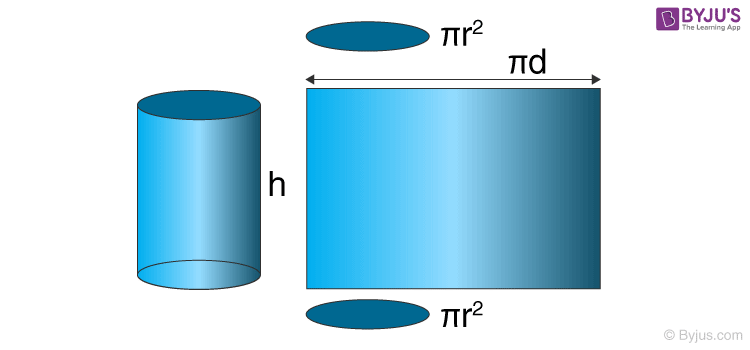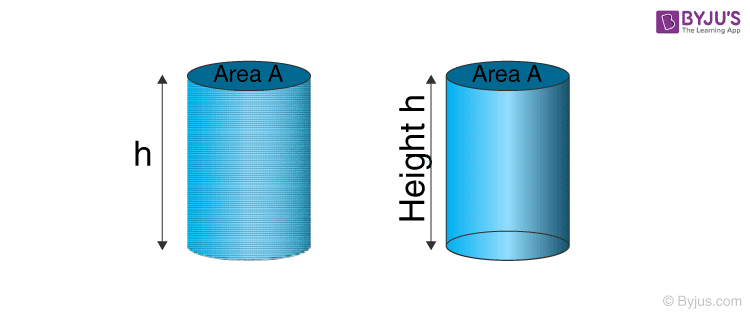# Volume Of A Cylinder Formula

The volume of a cylinder is used to measure the capacity of the cylinder and the amount of space occupied by it. To recall, a cylinder is a 3D structure that has two circular faces which are joined by a curved surface. A cylinder can be viewed as stacks of circular discs which are placed on one another. The total surface area of a cylinder can be calculated by summing up the areas of all its faces.

## Formula for Volume of a Cylinder

Mathematically, a cylinder’s volume can be expressed as:

 Volume of a Cylinder = πr2hFormula For Volume of a Cylinder

Where,

• h = The height of the cylinder

### Volume of a Cylinder Derivation:

As we mentioned, a cylinder is nothing but a set of circular discs stacked one upon the other. So if we compute the space occupied by each of these discs and add them up, what we get is the volume of the cylinder.

Let us say that the circular discs are stacked up to the height of ‘h’. Now, the volume of cylinder will be the product of the base area of the discs and the height ‘h’.Volume of the cylinder = Area of the circular base × Height

Area of the circular base = πr2
Height = h

Thus, the volume of a cylinder of height ‘h’ and base radius ‘r’ is given as πr2h.

Volume of a Cylinder = πr2h

### Applications of Cylinder’s Volume Formula in Real Life:

In our daily routine, we come across different types of situations where we have to handle cylindrical objects. The volume of cylinder formula is useful in calculating the capacity or volume of such cylindrical objects. This calculation can also help in designing cylindrical containers of larger or smaller volume as per the need. For example, the designing of-

• water tanks
• perfume bottles
• cylindrical containers
• cylindrical flasks used in chemistry labs, etc.

You can easily find out the volume of a cylinder if you have the measurements of its height and radius.

### Solved Example Questions

Question 1: The volume and height of a cylindrical container are 440 m³ and 35m respectively. Calculate its radius of the base. (Take π = 22/7)

Solution:

Given,

Volume of the cylindrical container = 440 m³

Height of the cylindrical container = 35 m

Volume of a Cylinder = πr2h

440 m³= πr2h

=>r2=440/ πh = (440 × 7 / 22 × 35)

=> r² = 4 m

=> r = 2 m

The radius of the base of the cylindrical container is 2 m.

Question 2: How many litres of water can a cylindrical water tank with base radius 20 cm and height 28 cm hold?

Solution: Given,

Base radius of the cylindrical water tank, r = 20 cm

Height of the cylindrical water tank, h = 28 cm

Volume of the cylindrical water tank = πr2h

Volume of the cylindrical water tank = 22/7 × 202× 28 = 35200 cm3

1 cubic centimeter = 0.001 litre =1 × 10-3 litre

∴ 35200 cubic centimeter = 35200 × 10-3 = 35.2 litres

The cylindrical water tank can hold 35.2 litres of water.